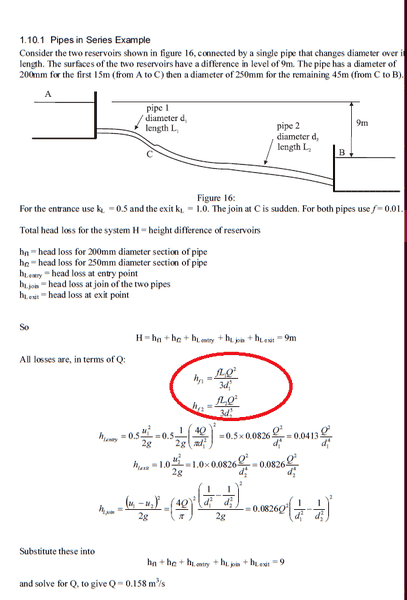# Major head loss in pipe

• foo9008
In summary, the authors use different equations to model the loss due to friction because the assumptions about how the world works affect the results.

## Homework Statement

why the author use major loss due to fluid friction is fL(Q^2) / 3(d^5) ?
according to darcy weisbech , the major loss due to friction has the formula of fL(v^2) / 2gD

why the author wrote it as fL(Q^2) / 3(d^5) ?
I don't understand## The Attempt at a Solution

since v= Q/ A , we can rewrite it as
fL(Q^2) / 2g(π^2)(d^5) , am i right ?

1. are the authors using the same models?
2. maybe (Q^2) / 3(d^5) = (v^2) / 2gD ?
... do you know what the variables mean and how the two formulas were derived, what assumptions they use?

since v= Q/ A , we can rewrite it as
fL(Q^2) / 2g(π^2)(d^5) , am i right ?
... that's the idea. Does this make the two equations the same?

•foo9008
Simon Bridge said:
1. are the authors using the same models?
2. maybe (Q^2) / 3(d^5) = (v^2) / 2gD ?
... do you know what the variables mean and how the two formulas were derived, what assumptions they use?

... that's the idea. Does this make the two equations the same?
what do you mean by same models here ?

ia my idea correct ? or the author's idea is correct ?

The mathematical equations you use are based on a set of assumptions about how the world works, which is described, in turn, by other maths.
The combinations of assumptions and maths is called a physical model.
Make different assumptions you get different equations.

You also get equations that look different but are actually the same ... for instance, the volume of a sphere could be written ##\frac{4}{3}\pi r^3## where ##r## is the radius, or it could be ##\frac{4}{3\sqrt{\pi}}A^{3/2}## ... where ##A## is the area of the biggest circular slice through the sphere, or it could be ##\frac{4}{16}\pi d^3## where ##d## is the diameter. You may use one equation instead of another because is is convenient to the information you have: i.e. it is easier to measure diameter than radius, but easier to build a sphere by fixing the radius rather than the diameter.

So do the two authors equation look different because they are actually different or is it more superficial than that?
The question is asking why the authors use the different equations ... that will be related to what they want to use the equations for as well as the assumptions they are making about reality.

I cannot tell if your idea is correct because I don't know what it is supposed to do. You didn't say.
However there is quite a lot going on which you have yet to address.